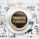TradingView1353 views
Return the average of simple moving averages with periods starting from min to max that is:

avg( sma ( src ,min), sma ( src ,min+1),..., sma ( src ,max))

The user can choose three types of weightings for the average, "simple", "linear", and "least squares".

Settings

• Min : minimum period of the sma
• Max : maximum period of the man, must be higher than "Min"
• Src : input data of the indicator
• Type : type of weighting, available options are "Simple", "Linear" or "Least Squares", by default "Simple"

Usage

The moving average can be used like any other classical moving average. The different types of weightings change the behavior of the moving average, the simple weighting will weight all the moving averages equally, a linear weighting will use the weighting function of a WMA , as such moving averages with lower periods will receive higher weights, this decrease the lag of the moving average. Finally, the least-squares weighting uses the weighting function of a least-squares moving average, this allows to drastically reduce the lag of the moving average.

in red the moving average using simple weighting, in blue linear weighting, and in orange least squares weighting, with all using min = 14 and max = 28.

In red the moving average with min = 50 and max = 200, in blue a LSMA of period 200, notice how the moving average has less overshoots.

Details

Computing the average of various simple moving averages is simple, remember that a simple moving average can be computed using a cumulative sum:

Sma = change(cum( src ),length)/length

we can't compute various "sma" functions with changing length argument within a for loop, but we can still differentiate within it, as such the cumulative sum method is super efficient and convenient.

The impulse response of this moving average is rectangular for the first "min" values, then the impulse is tailed, with the weighting method defining the shape of the tail.

in red the simple weighting method, in blue the linear method, and in orange the least-squares method.

Our moving average is an FIR moving average, as such the output lag is a linear characteristic of the moving average, which imply that:

Lag = Avg (lag( Sma (min)),lag( Sma (min+1))...,lag(max))

where lag is the lag of the moving average, in the case of a simple weighting we have:

Lag = Avg ((min-1)/2,(min+1-1)/2,...,(max-1)/2) = Avg ((min-1)/2,(max-1)/2)

a linear weighting gives a lag of:

Lag = Avg ((min-1)/3,(min+1-1)/3,...,(max-1)/3) = Avg ((min-1)/3,(max-1)/3)

Summary

A script computing the average of various moving averages has been presented, this MA might not be super useful to the everyday analyst but it stills have some great potential. Thx for reading.

This indicator is dedicated to my sister Lea, happy birthday kokoro
オープンソーススクリプト

TradingViewの精神に則り、このスクリプトの作者は、トレーダーが理解し検証できるようにオープンソースで公開しています。作者に敬意を表します！無料で使用することができますが、このコードの公開物での再利用には、ハウスルールに準拠する必要があります。お気に入りに登録してチャート上でご利用頂けます。

チャートでこのスクリプトを利用したいですか？
Patreon: https://www.patreon.com/alexgrover

Become a Patreon and get access to exclusive technical indicators!

You can also check out some of the indicators I made for luxalgo : https://www.tradingview.com/u/LuxAlgo/#published-scripts

## コメントThat's a very interesting idea there, will try with all the grocery list of MA's to see what happens, thanks! ^^Pratik_4Clover
@Pratik_4Clover, So many moving averages, so little time ^^ Thanks a lot for your support as always.